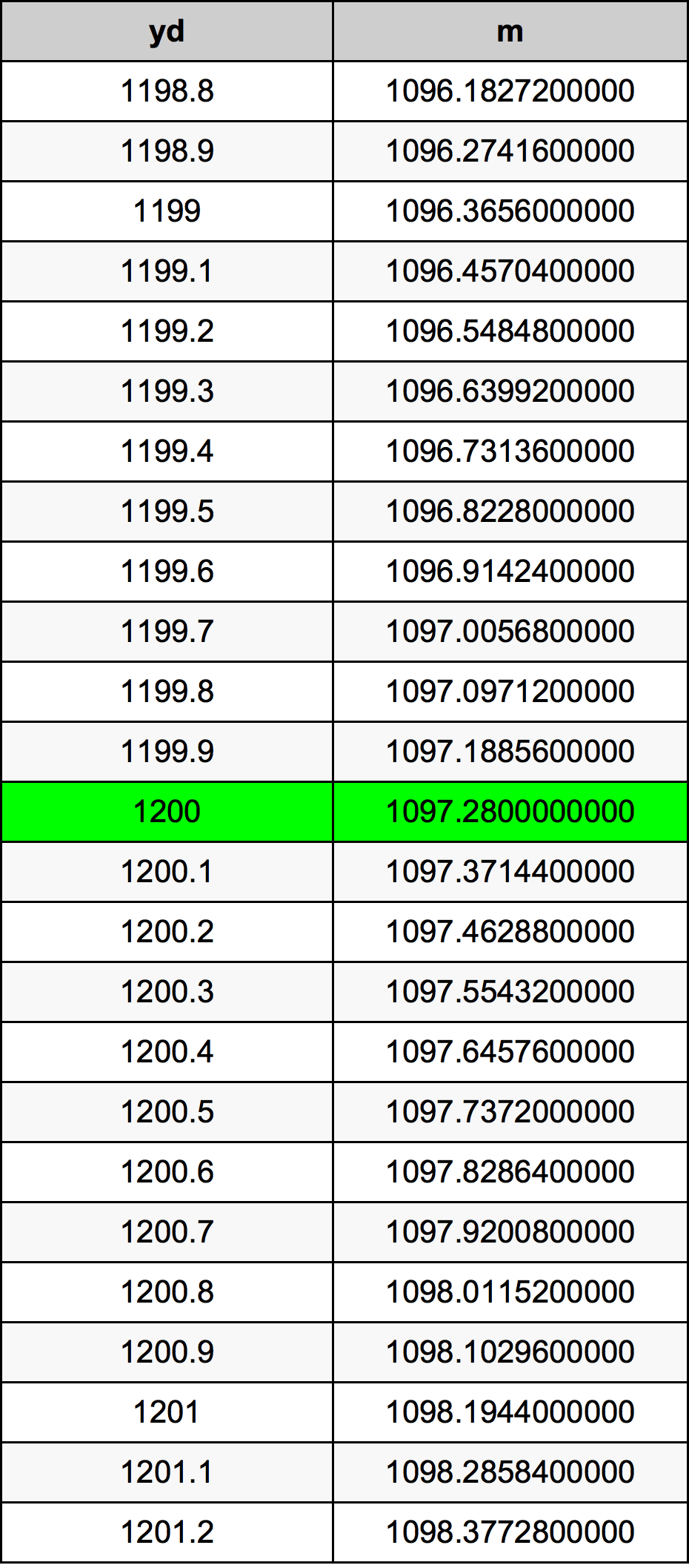Yards To Meters

# 1200 yd to m1200 Yards to Meters

yd
=
m

## How to convert 1200 yards to meters?

 1200 yd * 0.9144 m = 1097.28 m 1 yd
A common question is How many yard in 1200 meter? And the answer is 1312.33595801 yd in 1200 m. Likewise the question how many meter in 1200 yard has the answer of 1097.28 m in 1200 yd.

## How much are 1200 yards in meters?

1200 yards equal 1097.28 meters (1200yd = 1097.28m). Converting 1200 yd to m is easy. Simply use our calculator above, or apply the formula to change the length 1200 yd to m.

## Convert 1200 yd to common lengths

UnitLength
Nanometer1.09728e+12 nm
Micrometer1097280000.0 µm
Millimeter1097280.0 mm
Centimeter109728.0 cm
Inch43200.0 in
Foot3600.0 ft
Yard1200.0 yd
Meter1097.28 m
Kilometer1.09728 km
Mile0.6818181818 mi
Nautical mile0.5924838013 nmi

## What is 1200 yards in m?

To convert 1200 yd to m multiply the length in yards by 0.9144. The 1200 yd in m formula is [m] = 1200 * 0.9144. Thus, for 1200 yards in meter we get 1097.28 m.

## 1200 Yard Conversion Table## Alternative spelling

1200 yd to Meters, 1200 yd in Meters, 1200 Yards to m, 1200 Yards in m, 1200 Yard to Meters, 1200 Yard in Meters, 1200 yd to Meter, 1200 yd in Meter, 1200 Yard to Meter, 1200 Yard in Meter, 1200 Yards to Meter, 1200 Yards in Meter, 1200 Yards to Meters, 1200 Yards in Meters